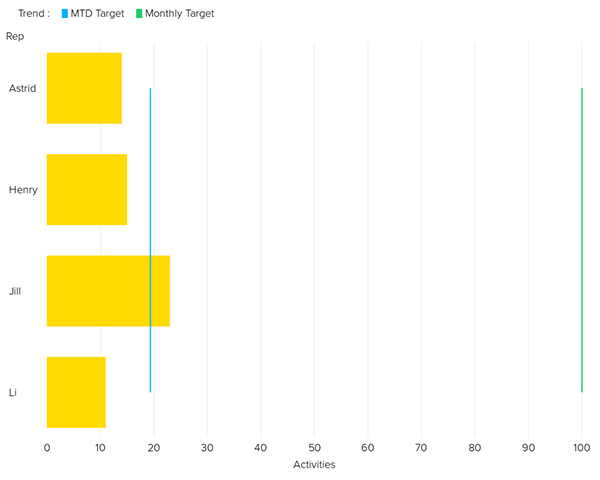Creating run rate calculations

Follow

To be able to compare results against pro-rated targets, you need to know where you are in the month and how far there still is to go. In the calculation engine, you can create calculated metrics to identify the number of days.

There are two standard calculated metrics you can use to create this comparison:

Days elapsed

To create a metric for how many days have elapsed during a time period, follow the steps below.

1. In the Query Builder, open the Calculation engine menu .
2. Select the Standard calculated metric option.
3. Enter in the following formula:
` DAY(TODAY())`
4. If you are working with weekly targets, you can enter in the following formula to calculate the number of days elapsed in a working week starting on Sunday:
` WEEKDAY_NUMERIC(TODAY())`
5. For a working week starting on a Monday, you can use the following formula:
`WEEKDAY_NUMERIC(TODAY())-1`
6. When you have finished entering your formula, click Save .

Days in Month

To create a metric for how many days there are in total in a time period, please follow the steps below.

1. In Calculation engine menu > Standard calculated metric enter in the following formula:
`DAY(END_OF_MONTH(TODAY()))`
2. Click the Save button.
3. Click the + next to Metrics, and select your calculated metrics from the drop-down list.

You can then create queries for reports like:

• Pro-rate targets.
• Calculate your current run rate.
• Anticipate your end of month results.
• Calculate what is needed to make up any shortfall.

For example, these calculated metrics can be used to monitor sales activity to see who was on or off target.